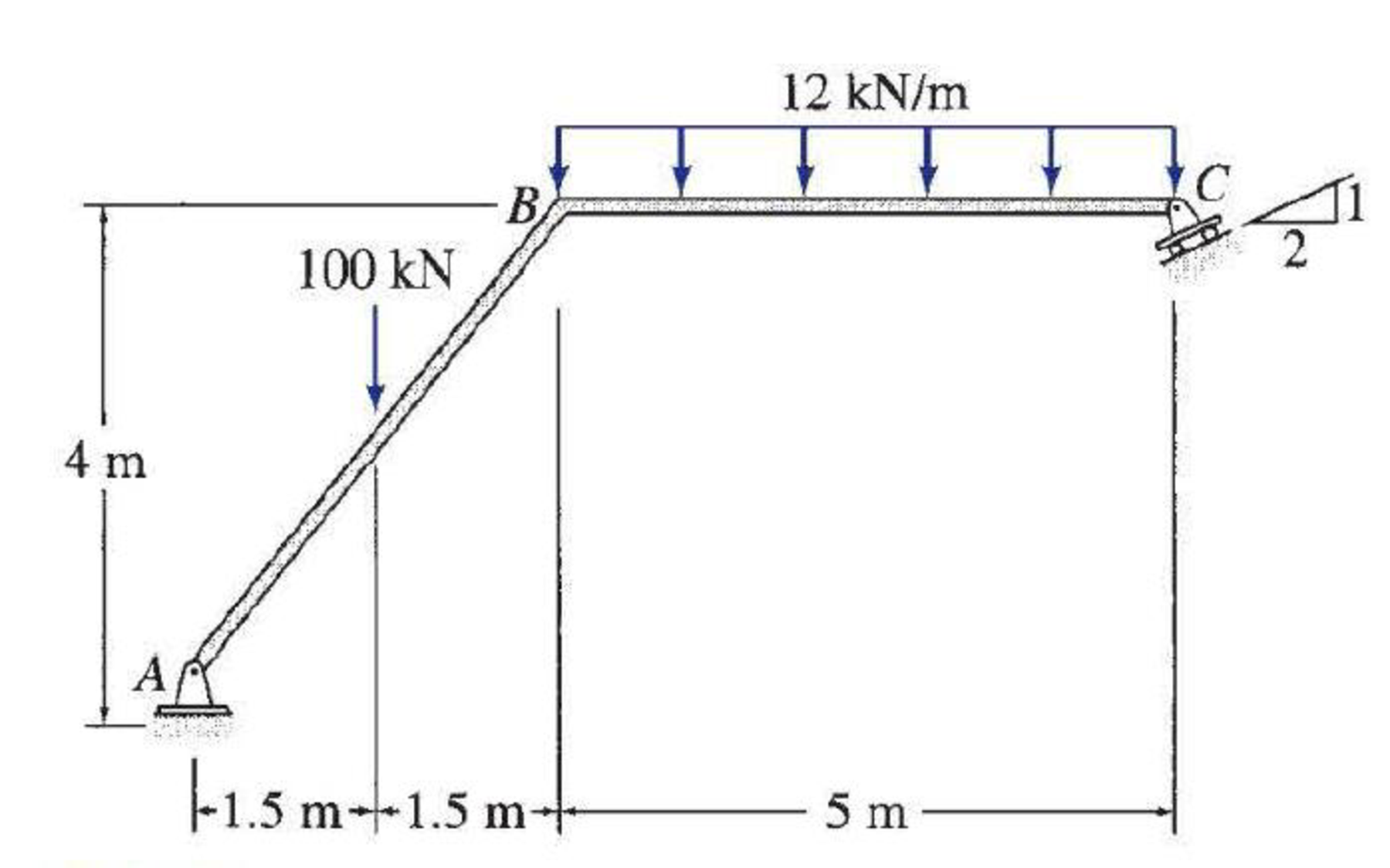# Draw the shear, bending moment, and axial force diagrams and the qualitative deflected shape for the frame shown.

#### Solutions

Chapter
Section
Chapter 5, Problem 59P
Textbook Problem
778 views

## Draw the shear, bending moment, and axial force diagrams and the qualitative deflected shape for the frame shown.To determine

Plot the shear diagram, bending moment diagram, axial force diagram, and the qualitative deflected shape of the frame.

### Explanation of Solution

Write the condition for static instability, determinacy and indeterminacy of plane frames as follows:

3m+r<3j+ecstaticallyunstableframe        (1)

3m+r=3j+ecstaticallydeterminateframe        (2)

3m+r>3j+ecstaticallyindeterminateframe        (3)

Here, number of members is m, number of external reactions is r, the number of joints is j, and the number of elastic hinges is ec.

Find the degree of static indeterminacy (i) using the equation;

i=(3m+r)(3j+ec)        (4)

Refer to the Figure in the question;

The number of members (m) is 2.

The number of external reactions (r) is 3.

The number of joints (j) is 3.

The number of elastic hinges ec is 0.

Substitute the values in Equation (2);

3(2)+3=3(3)+09=9staticallydeterminateframe

Show the free-body diagram of the entire frame as in Figure 1.

Find the resultant reaction at point C by taking moment about point A.

+MA=0100(1.5)12(5)(52+3)+RC(15)(4)+RC(25)(8)=0150330+4RC5+16RC5=0RC=53.67kN

Consider point C;

Find the vertical reaction at point C by resolving the vertical component of forces.

+FYC=0Cy=RC(25)=53.67(25)=48kN

Consider point C;

Find the horizontal reaction at point C by resolving the horizontal component of forces.

+FXC=0Cx=RC(15)=53.67(15)=24N

Show the free-body diagram of the members and joints of the entire frame as in Figure 2.

Consider point C:

Resolve the vertical component of forces.

+FY=048CYBC=0CYBC=48kN

Resolve the horizontal component of forces.

+FX=0CXBC24=0CXBC=24kN

Consider the member BC:

Resolve the vertical component of forces.

+FY=0BYBC+CYBC12(5)=0BYBC+4860=0BYBC=12kN

Resolve the horizontal component of forces.

+FX=0BXBCCXBC=0BXBC24=0BXBC=24kN

Take moment about the point B

### Still sussing out bartleby?

Check out a sample textbook solution.

See a sample solution

#### The Solution to Your Study Problems

Bartleby provides explanations to thousands of textbook problems written by our experts, many with advanced degrees!

Get Started

Find more solutions based on key concepts
Perform a Web search to obtain information about the number of engineers employed by specific area and their me...

Engineering Fundamentals: An Introduction to Engineering (MindTap Course List)

Check the adequacy of the bolts. The given loads are service loads. a. Use LRFD. b. Use ASD.

Steel Design (Activate Learning with these NEW titles from Engineering!)

Define the term, Ethernet. Explain what happens when two devices on an Ethernet attempt to send data at the sam...

Enhanced Discovering Computers 2017 (Shelly Cashman Series) (MindTap Course List)

What is the difference between a threat agent and a threat?

Principles of Information Security (MindTap Course List)

Identify and briefly describe five common fact-finding methods.

Systems Analysis and Design (Shelly Cashman Series) (MindTap Course List)

List three safety precautions to be observed when using a hacksaw.

Precision Machining Technology (MindTap Course List)

If your motherboard supports ECC DDR3 memory, can you substitute non-ECC DDR3 memory?

A+ Guide to Hardware (Standalone Book) (MindTap Course List)